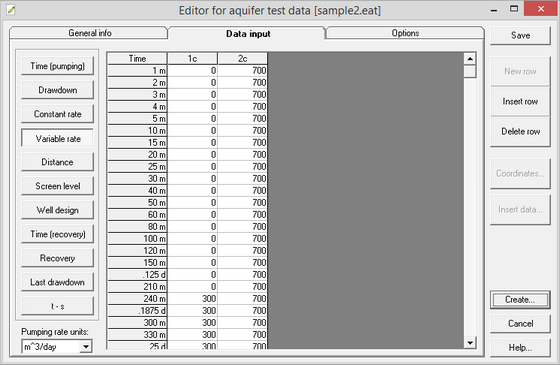﻿ ANSDIMAT+ > Data input (Editor) > Table data input > Variable rate

Variable rate

Navigation:  ANSDIMAT+ > Data input (Editor) > Table data input >

Variable rate

Input variable rate for all pumping wells for times listed in table "Time". This table is used when interpretation is conducted with variable pumping rates. Rate dimension is selected from the combo box "Pumping rate units". For data processing the rate is automatically converted by the program to cubic meters per day.Dialog box for aquifer test data input: variable rate.

Explanatory table

 "Time" column Time, automatically copied from the "Time" table. The time value is followed by the unit: d – day, h – hour, m – minute, s – second. For data processing, time is automatically converted to days Columns with names of pumping wells Variable pumping rates for all times in the “Time” table. Each column corresponds to a pumping well "Insert row" button Moves pumping rate values one line down only for the selected pumping well. The number of observation points doesn’t change so the whole time series is shifted down one step from the inserted row and the entry corresponding to the last time is lost "Delete row" button Delete the current value and move the rate value one line up only for the selected pumping well. The number of observation points doesn’t change so the whole time series is shifted up one step from the deleted row and the entry corresponding to the last time is blank "Pumping rate units" combo box Select rate unit: m3/day, m3/hour, m3/min, m3/sec, l/day, l/hour, l/min, l/sec. When units are modified, ANSDIMAT automatically recalculates the pumping rate in cubic meters per day for data processing